• python while循环语句用法
千次阅读
2021-06-23 15:38:26

#### 1.while 循环结构

特征:减少代码冗余,提升代码效率

语法:

while 条件表达式:
code1
code2
...


1.初始化一个变量

2.写上循环的条件表达式

3.自增自减的变量值

案例1:打印1~100

i = 1
while i<=100:
# 要执行的逻辑 ...
print(i)
i += 1

"""
初始化一个变量 i

第一次循环:
i <= 100 条件成立,执行循环
直接打印 print(i) => 1
i += 1 => i = 2

第二次循环:
回到17行,重新回到判断,看一看是否满足条件
2 <= 100 条件成立,执行循环
直接打印 print(i) => 2
i += 1 => i = 3

第三次循环:
回到17行,重新回到判断,看一看是否满足条件
3 <= 100 条件成立,执行循环
直接打印 print(i) => 3
i += 1 => i = 4

...
...
依次类推进行循环 ...

到什么时候结束?
当打印100 结束之后
i += 1 => i = 101 回到循环的判断条件中
发现101 <= 100 不满足条件,不成立,不执行循环体代码块
到此为止,循环直接结束.

"""


案例2 : 打印1~100的累加和?1+2+3+4+ …+100 ?

#方法一
total 负责做累加和
total = 0
i = 1
while i<=100:
total += i
i+=1

print(total)

"""
# 代码解析:

第一次循环:
1 <= 100 条件为真 , 执行循环
total += i => total = total + i => total = 0 + 1
i+=1 => i=2

第二次循环:
回到条件表达式中进行判断
2<=100  条件为真 , 执行循环
total += i => total = total + i => total = 0 + 1 + 2
i+=1 => i=3

第三次循环:
回到条件表达式中进行判断
3<=100  条件为真 , 执行循环
total += i => total = total + i => total = 0 + 1 + 2 + 3
i+=1 => i=4

...
...
依次循环

什么时候结束?
total = 0 + 1 + 2 + 3 + ... + 100
i+=1 => i = 101
回到条件表达式中进行判断
101 <= 100 条件不成立,不执行循环体;
直接终止循环;

print(total)  5050
"""
'''
Python学习交流，免费公开课，免费资料，
免费答疑，系统学习加QQ群：579817333
'''

# 方法二
# 1+2+3+4+ .....+100 ? 用while True 的方式实现;
total = 0
i = 1
sign = True
while sign:
total += i
i+=1

# 手动加上终止的条件
if i == 101:
sign = False

print(total)
# 这种情况下就不执行循环了;用这样的方式终止循环;
while False:
print(111)


#### 2.死循环

while True:
print(123)


(1.单循环练习:

1.打印一行十个小星星

i = 1
while i <= 10:
print("★",end="")
i += 1


2.用一个变量打印出一行十个小星星

'''
Python学习交流，免费公开课，免费资料，
免费答疑，系统学习加QQ群：579817333
'''

i = 0
strvar = ""
while i<10:
# 循环10次,拼接10个星星,每循环一次拼接一个星星
strvar += "★"
i+=1
print(strvar)


3.打印一行十个小星星 奇数个打印★ 偶数个打印☆

i = 1
while i <= 10:
if i % 2 == 0:
print("☆",end='')
else:
print("★",end="")
i += 1

#方法二
print("-----分割线-------")
count = 1
str_var = ""
while count <= 10:
if count % 2 == 0:
str_var += "☆"
else:
str_var += "★"
count += 1
print(str_var)


4.一个循环,打印十行十列小星星

#循环100和星星
i = 1
while i <= 100:
#1.打印小星星
print("★",end='')
#2.打印换行
if i % 10 == 0:
print()
i += 1


5.一个循环 打印十行十列隔列变色小星星

#方法一
i = 0
while i < 100:
if i % 2 == 0:
print("☆",end="")
else:
print("★",end='')
if i % 10 == 9:
print()
i += 1

#方法二
_str_var = ""
count = 1
while count <= 100:
if count % 2 == 0:
_str_var += "☆"
else:
_str_var += "★"
if count % 10 == 0:
_str_var +="\n"
count += 1
print(_str_var)


6.一个循环 打印十行十列隔行变色小星星

'''
Python学习交流，免费公开课，免费资料，
免费答疑，系统学习加QQ群：579817333
'''

i = 0
while i < 100:
#打印星星
if i // 10 %2 == 0:
print("☆", end="")
else:
print("★",end="")
if i % 10 == 9:
print()
i += 1


(2.pass break continue

1.pass 过 (当不能直接在代码块中写上具体代码时,先用pass占位;)

num = 10
if num == 10:
pass

i = 0
while i<10:
pass
i+=1


2.break 终止当前循环,只能用在循环当中

# 打印1~10 ,遇到5就终止循环
# 单循环 (终止当前循环)
i = 1
while i<=10:

if i == 5:
break

print(i)

i+=1


多循环 (终止当前循环)

i = 0
while i<3:
j = 0
while j<3:
if j ==2:
break
print(i,j)
j+=1
i+=1
# 不能再循环外使用break
"""
if 5==5:
print(111)
break
"""


3.continue 跳过当前循环,从下一次开始 ,只能用在循环当中

打印1 ~ 10 不包括5

i = 1
while i<=10:

if i == 5:
# 手动加1 , 防止跳过下面代码,忽略自增形成死循环;
i+=1
continue
print(i)
i+=1


打印1~100 所有不含有4的数字;

"""
// 地板除可以取一个数的高位
%  取余  可以取一个数的低位

任意数 // n 会产生n个相同的数字
任意数 %  n 取值范围是0~(n-1)
"""
# 方法一
i = 1
while i<=100:

if i % 10 == 4 or i // 10 == 4:
# 手动自增1,防止死循环
i+=1
continue
print(i)
i+=1

# 方法二 in
i = 1
while i<=100:
strvar = str(i)
if '4' in strvar:
i+=1
continue

print(i)
i+=1


(3.双层循环练习

1.十行十列小星星

'''
Python学习交流，免费公开课，免费资料，
免费答疑，系统学习加QQ群：579817333
'''

#j动1次,i动10次,外层循环动的慢.内层循环动的快
j = 0
while j<10:
#打印星星
i = 0
while i < 10:
print("*",end='')
i += 1
# 打印换行
print()

j += 1


2 双层 十行十列隔列换色小星星

j = 0
while j<10:
#打印一行十个星星
i = 0
while i < 10:
if i % 2 == 0:
print("☆",end='')
else:
print("★",end='')
i += 1
#打印一个换行
print()
j += 1


3双层 十行十列隔行换色小星星

"""
当j = 0 时,在内存循环,循环了10次 ☆
当j = 1 时,在内层循环,循环了10次 ★
"""
print("------分割线------")
j = 0
while j < 10:
#打印一行十个小星星
i = 0
while i < 10:
if j % 2 == 0:
print("★",end='')
else:
print("☆",end='')
i += 1
#打印一个换行
print()
j += 1


4.打印99乘法表

"""
外层的i控制行 里层的j控制列
当前j跟当前行数有关系,不能大于当前行 所以j <= i
"""
#方法一(正序 1~9)
i = 1
while i <= 9:
j = 1
while j <= i:
#字符串的格式化 让最后的乘积占用2位,据右边显示;
print("%s*%s =%2s"%(i,j,i*j),end=" ")
j += 1
print()
i += 1
"""
'字符串'%(值1,值2,值3,......)
%s 字符串占位符
res = "%s * %s = ?"% (5,6)
print(res)
"""
# 方法二(倒序 9 ~1)
i = 9
while i >= 1:
#字符串的格式化 让最后的乘积占用2位,据右边显示;
j = 1
while j <= i:
#字符串的格式化 让最后的乘积占用2位,据右边显示;
print("%d*%d=%2d"% (i,j,i*j),end=' ')
j += 1
#打印换行
print()
i -= 1

# 方法三
i = 1
while i <= 9:
#(1)先打印空格
k = 9 - i
while k > 0:
#	"""打印空格"""
print("      ",end="")
k -= 1

#print("       "*(9-i),end="")
#(2)打印表达式
j = 1
while j <= i:
print("%d*%d=%2d"% (i,j,i*j),end="")
j += 1
#(3)打印换行
print()
i +=1

print("-----分割线-----")
#方法四
i = 9
while i > 0:
k = 9 - i
while k > 0:
print("         ",end='')
k -= 1
# print("       "*(9-i),end='')
# (2) 打印表达式
j = 1
while j <= i:
#字符串的格式化 让最后的乘积占用2位,据右边显示;
print("%d*%d = %2d"% (i,j,i*j),end=' ')
j += 1
#打印换行
print()
i -= 1


5 求吉利数字100 ~ 999 666 888 111 222 333 444 … 123 789 567 987 321 543 210

"""
// 地板除可以获取一个数的高位
% 取余 可以获取一个数的低位

98 98 % 10 => 8
98 98 // 10 => 9

678
个位 = 678 % 10 => 8
十位 = 678 // 10 %10 => 7
百位 = 678 // 100 => 6
"""
#方法一
i = 100
while i <= 999:
gewei = i % 10
shiwei = i // 10 % 10
baiwei = i // 100

#gewei = shiwei = baiwei 三者相同
if shiwei == gewei and shiwei ==baiwei:
print(i)

#678
if shiwei == gewei -1 and shiwei == baiwei + 1:
print(i)

#876
if shiwei == gewei + 1 and shiwei == baiwei - 1:
print(i)
i += 1

#方法二 字符串形式写
print("-----分割线-------")
i = 100
while i<= 999:
num = str(i)
#"987"
geiwei = int(num[-1]) #7
shiwei = int(num) #8
baiwei = int(num) #9

if shiwei == gewei and shiwei == baiwei:
print(i)

if shiwei == gewei - 1 and shiwei == baiwei + 1:
print(i)

if shiwei == gewei + 1 and shiwei == baiwei - 1:
print(i)

i += 1


#### 经典题: 百钱买百鸡

公鸡,母鸡,小鸡,公鸡1块钱一只,母鸡3块钱一只,小鸡是5毛钱一只,问100块钱买100只鸡,有多少种买法
穷举法: 一个一个拿出来试

x [1,2]
y [3,4]
z [5,6]


满足 x+y+z = 10

1 + 3 + 5 = 9
1 + 3 + 6 = 10 ok

1 + 4 + 5 = 10 ok
1 + 4 + 6 = 11

2 + 3 + 5 = 10 ok
2 + 3 + 6 = 11

2 + 4 + 5 = 11
2 + 4 + 6 = 12


公鸡x只,母鸡y只,小鸡z只

x+y+z = 100 #一百只鸡
x+3+y*1+0.5*z = 100


两个条件同时满足

x = 0
total = 0
while x<=100:
y = 0
while y<= 33:
z = 0
while z <= 100:
if(x+y+z == 100) and (x+3*y+0.5*z == 100):
total += 1
z += 1
y += 1
x += 1
print(total)python
更多相关内容
• 主要介绍了pythonwhile循环语句用法,以一个简单实例形式分析了Python使用while循环语句使用方法,需要的朋友可以参考下
• Python 语言中用来控制循环的主要有两个句法，while 和 for 语句，本节将简单介绍 while 句法的使用。 2.while结构 while 语句同其他编程语言中 while使用方式大同小异，主要结构如下 while condition: #当...
• 1.while语句的语法及用法 （1）语法 while <condition>条件语句: <execute statement>执行语句 参数说明 condition：为条件语句，用来设置循环的条件，可为等于、比较等。比如a==3、a>2。当...

1.while语句的语法及用法

（1）语法

while <condition>条件语句:
<execute statement>执行语句

参数说明

condition：为条件语句，用来设置循环的条件，可为等于、比较等。比如a==3、a>2。当条件为TURE即while true表示无限循环。

execute statement：执行语句，即满足循环条件之后需要执行什么。

（2）用法：用于条件或无限循环。

2.实例

（1）条件循环

例：一个变量为5，当i>2时输出i-1的值。

#while循环
i =5  #定义i
while i>2:
i = i-1  #满足while条件则i减1
print(i) #输出i值
#当i<=2时停止输出

（2）无限循环

①与条件语句以及input函数结合使用，实现循环判断语句。

例：无限输入成绩数值判断成绩等级。

while True: #循环
score = int(input('输入数值：'))  #输入
#条件判断
if score >= 90:
print('优秀')
elif score >=80:
print('良好')
elif score >= 70:
print('一般')
elif score >= 60:
print('及格')
else:
print('不及格')

运行结果如下图所示。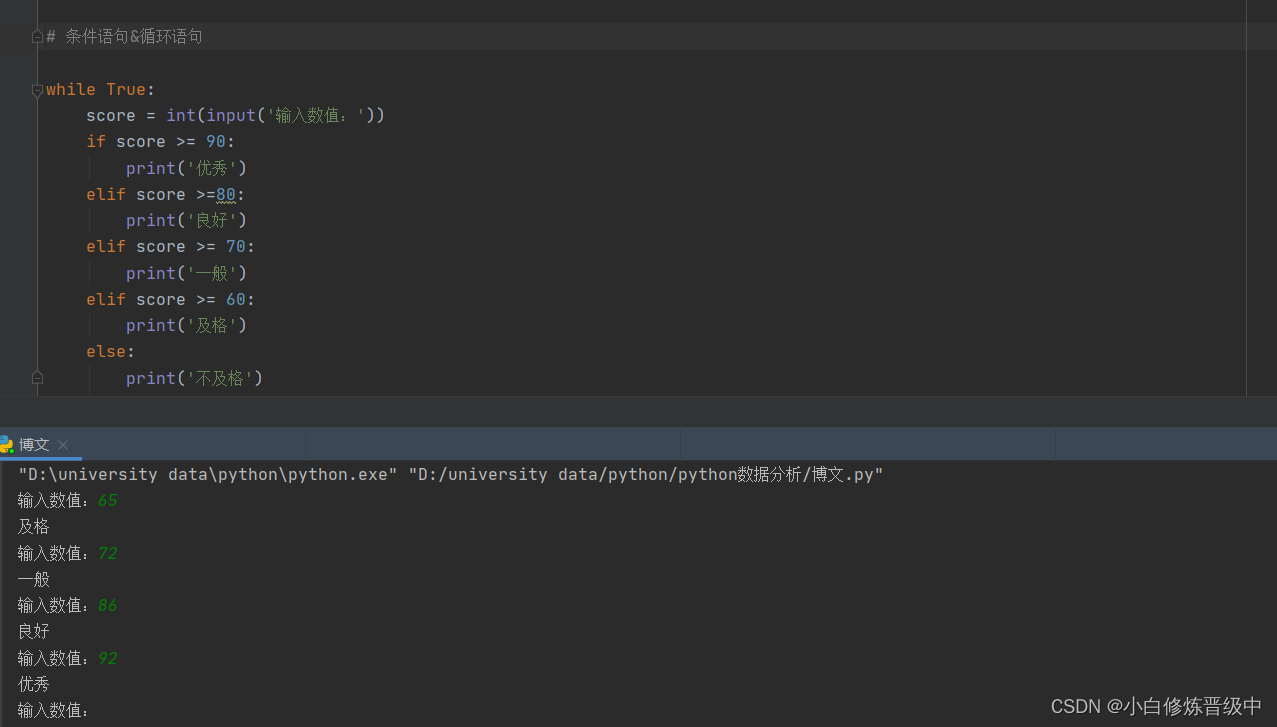if条件语句具体用法实例可参考：https://blog.csdn.net/weixin_50853979/article/details/124975196

input函数具体用法实例可参考：https://blog.csdn.net/weixin_50853979/article/details/124978009

展开全文python 开发语言 经验分享
• Python while循环语句详解 Python 中，while 循环和 if 条件分支语句类似，即在条件（表达式）为真的情况下，会执行相应的代码块。不同之处在于，只要条件为真，while 就会一直重复执行那段代码块。 while 语句的...

# Python while循环语句详解

Python 中，while 循环和 if 条件分支语句类似，即在条件（表达式）为真的情况下，会执行相应的代码块。不同之处在于，只要条件为真，while 就会一直重复执行那段代码块。

while 语句的语法格式如下：

while 条件表达式：
代码块


这里的代码块，指的是缩进格式相同的多行代码，不过在循环结构中，它又称为循环体。

while 语句执行的具体流程为：首先判断条件表达式的值，其值为真（True）时，则执行代码块中的语句，当执行完毕后，再回过头来重新判断条件表达式的值是否为真，若仍为真，则继续重新执行代码块…如此循环，直到条件表达式的值为假（False），才终止循环。

while 循环结构的执行流程如图 1 所示。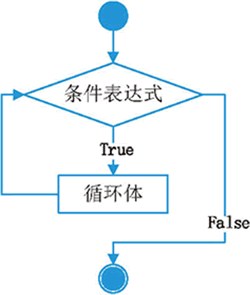图 1 while 循环语句执行流程示意图

例如，打印 1~100 的所有数字，就可以使用 while 循环，实现代码如下：

# 循环的初始化条件
num = 1
# 当 num 小于100时，会一直执行循环体
while num < 100 :
print("num=", num)
# 迭代语句
num += 1
print("循环结束!")


运行程序会发现，程序只输出了 1~99，却没有输出 100。这是因为，当循环至 num 的值为 100 时，此时条件表达式为假（100<100），当然就不会再去执行代码块中的语句，因此不会输出 100。

注意，在使用 while 循环时，一定要保证循环条件有变成假的时候，否则这个循环将成为一个死循环。所谓死循环，指的是无法结束循环的循环结构，例如将上面 while 循环中的num += 1代码注释掉，再运行程序你会发现，Python 解释器一直在输出"num= 1"，永远不会结束（因为 num<100 一直为 True），除非我们强制关闭解释器。

再次强调，只要位于 while 循环体中的代码，其必须使用相同的缩进格式（通常缩进 4 个空格），否则 Python 解释器会报 SyntaxError 错误（语法错误）。例如，将上面程序中num+=1语句前移一个空格，再次执行该程序，此时 Python 解释器就会报 SyntaxError 错误。

除此之外，while 循环还常用来遍历列表、元组和字符串，因为它们都支持通过下标索引获取指定位置的元素。例如，下面程序演示了如何使用 while 循环遍历一个字符串变量：

my_char="http://www.baidu.com/"
i = 0;
while i<len(my_char):
print(my_char[i],end="")
i = i + 1


程序执行结果为：
http://www.baidu.com/

展开全文Python循环语句 while
• 主要介绍了Python While循环语句实例演示及原理解析,文中通过示例代码介绍的非常详细，对大家的学习或者工作具有一定的参考学习价值,需要的朋友可以参考下
• 本文实例讲述了python循环语句while用法。分享给大家供大家参考。具体分析如下： 对于pythonwhile语句,注意其缩进即可。 python和其他语言一样也有break和continue，分别用来表示跳出循环和继续循环。 #!/usr/...
• Python 编程中 while 语句用于循环执行程序，即在某条件下，循环执行某段程序，以处理需要重复处理的相同任务。其基本形式为： while 判断条件(condition)： 执行语句(statements)…… 执行语句可以是单个语句语句...

Python 编程中 while 语句用于循环执行程序，即在某条件下，循环执行某段程序，以处理需要重复处理的相同任务。其基本形式为：

while  判断条件(condition)：  执行语句(statements)……

执行语句可以是单个语句或语句块。判断条件可以是任何表达式，任何非零、或非空（null）的值均为true。

当判断条件假 false 时，循环结束。

执行流程图如下：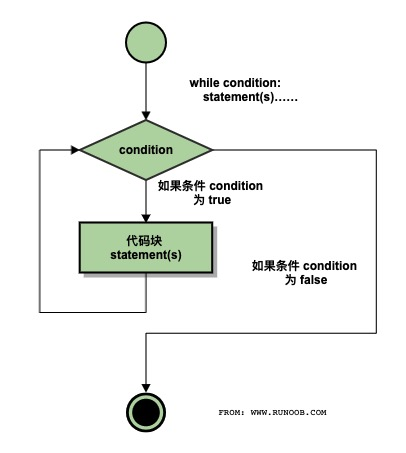### Gif 演示 Python while 语句执行过程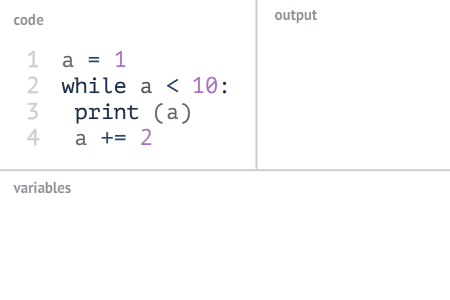复杂一点: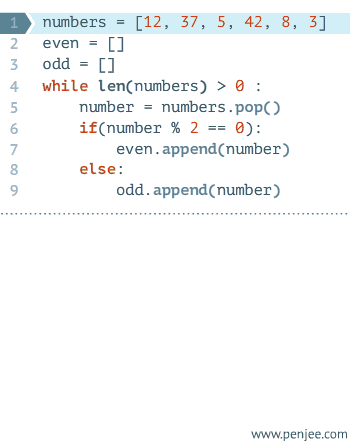## 实例

#!/usr/bin/python
count = 0
while  (count < 9):
print  'The count is:',
count  count = count + 1
print  "Good bye!"


以上代码执行输出结果:

The count is:  0  The count is:  1  The count is:  2  The count is:  3  The count is:  4  The count is:  5  The count is:  6  The count is:  7  The count is:  8  Good bye!

while 语句时还有另外两个重要的命令 continue，break 来跳过循环，continue 用于跳过该次循环，break 则是用于退出循环，此外"判断条件"还可以是个常值，表示循环必定成立，具体用法如下：

# continue 和 break 用法
i = 1  while  i < 10: i += 1  if  i%2 > 0:
# 非双数时跳过输出  continue
print  i
# 输出双数2、4、6、8、10
i = 1
while  1:
# 循环条件为1必定成立
print  i
# 输出1~10  i += 1
if  i > 10:
# 当i大于10时跳出循环
break


## 无限循环

如果条件判断语句永远为 true，循环将会无限的执行下去，如下实例：

## 实例

#!/usr/bin/python # -- coding: UTF-8 -- var = 1 while var == 1 : # 该条件永远为true，循环将无限执行下去 num = raw_input(“Enter a number :”) print "You entered: ", num print “Good bye!”

以上实例输出结果：

Enter a number :20  You entered:  20  Enter a number :29  You entered:  29  Enter a number :3  You entered:  3  Enter a number between :Traceback  (most recent call last):  File  "test.py", line 5,  in   num = raw_input("Enter a number :")  KeyboardInterrupt

**注意：**以上的无限循环你可以使用 CTRL+C 来中断循环。

## 循环使用 else 语句

在 python 中，while … else 在循环条件为 false 时执行 else 语句块：

## 实例

#!/usr/bin/python count = 0 while count < 5: print count, " is less than 5" count = count + 1 else: print count, " is not less than 5"

以上实例输出结果为：

0  is less than 5  1  is less than 5  2  is less than 5  3  is less than 5  4  is less than 5  5  is  not less than 5

## 简单语句组

类似 if 语句的语法，如果你的 while 循环体中只有一条语句，你可以将该语句与while写在同一行中， 如下所示：

## 实例

#!/usr/bin/python flag = 1 while (flag): print ‘Given flag is really true!’ print “Good bye!”

**注意：**以上的无限循环你可以使用 CTRL+C 来中断循环。

python福利教程领取方式：
1、点赞+评论（勾选“同时转发”）
2、关注小编。并私信回复关键字【19】
（一定要私信哦~点击我的头像就能看到私信按钮了）

展开全文python 开发语言 后端
• 本文实例讲述了Python基础之循环语句用法。分享给大家供大家参考，具体如下： while 循环 Python中while语句的一般形式： while 判断条件：  statements 同样需要注意冒号和缩进。另外，在Python中没有do..while...
• Python中，while 循环和 if 条件分支语句类似，即在条件(表达式)为真的情况下，会执行相应的代码块。不同之处在于，只要条件为真，while 就会一直重复执行那段代码块。while 语句的语法格式如下：while 条件表达式：...
• Python循环语句的逻辑：执行一个语句/一段代码块多次。简单理解，循环就是反复的去做某一件事情。 循环结构： while 表达式1： 代码1 当表达式为真时，则运行代码1，然后回到表达式再进行判断是否为真，重复这...python
• Python 编程中 while 语句用于循环执行程序，即在某条件下，循环执行某段程序，以处理需要重复处理的相同任务。其基本形式为： while 判断条件： 执行语句…… 执行语句可以是单个语句语句块。判断条件可以是...
• 表达的是一个逻辑表达式，必须返回一个true或false值，本文章向码农介绍Python while 循环使用方法，需要的朋友可以看一下本文章。 一个循环是一个结构，导致第一个程序要重复一定次数。重复不断循环的条件仍是如此...
• (有编程基础的同学要注意，在Python while循环语句中，是没有do...while格式的循环语句的)Python作为后台语言，在其他语言的基础上，取其精华去其糟粕：单从循环语句这一点来看，它所使用的，在其他语言中都是不可...
• Python循环终止语句有三种： 1、break break用于退出本层循环 示例如下： while True: print 123 break print 456 2、continue continue为退出本次循环，继续下次循环 示例如下: while True: print 123 ...
• python中的循环语句主要有while循环和for循环两种。本文主要讲while循环，下一篇文章将继续for循环的讲解。1while 循环语句结构while 条件表达式:循环体语句块while循环中，当条件表达式为真时...
• while循环语句和if条件语句一样，需要注意冒号(:)和缩进，Python3中没有do…while语句 a、形式 while 判断条件(condition)： 执行语句(statements)…… b、流程图 c、无限循环 如果while循环语句的条件表达式结果...
• 只要给定条件为真(True)，Python编程语言中的while循环语句将重复执行目标语句。语法Python编程语言中的while循环的语法是 -while expression:statement(s)在这里，语句(statement(s))可以是一个单一的语句或一组...
• while循环基础 while循环练习 while循环嵌套 一、while循环基础 while语句的基本语法： 初始条件设置 —— 通常是重复执行的 计数器 while 条件（判断 计数器 是否达到 目标次数）： 条件满足时，做的事情1 ...python
• 1.while循环基本使用 循环的作用就是让指定的代码重复的执行 . while循环最常用的应用场景就是让执行的代码按照指定的次数重复执行 需求--打印5遍Hello World 思考--如果要求打印100遍怎么办? 1.1 while语句...
• ## Python中while循环的基本用法

万次阅读 多人点赞 2019-09-11 10:21:34
while和for都可以使程序重复执行某一段代码（又称作循环体），区别是for循环用于针对集合中的每一个元素都一个代码块，而while循环在表达式为True的情况下会不断地执行，直到指定条件不满足为止。 今天我们一起来...python 循环 while循环 基础教程
• 本篇介绍Python循环语句的使用，文中主要讨论while循环语句使用while循环语句可以解决程序中需要重复执行的操作，本文讲解的每个案例中都配有流程图和代码说明。通过本篇的学习，可以达成如下目标。● 掌握循环...
• python循环语句——while循环前言 前言 Python中除了for循环之外，还有一个while循环。虽然都是循环，for循环主要用于遍历，但是while循环主要用于循环执行程序，即在满足某个条件下，循环执行某段程序，以处理需要...python pycharm
• 程序在一般情况下是按顺序执行的。编程语言提供了各种控制结构，允许更复杂的执行路径。 循环语句允许我们执行一个语句或语句组多次，下面是...在了解循环语句使用方法之前，我们先来了解几个循环控制语句： 1）...
• python使用while循环的实例python中for循环用于针对集合中的每个元素的一个代码块，而while循环能实现满足条件下的不断运行。使用while循环时，由于while只要满足条件就会执行代码，故必须指定结束条件，否则会......